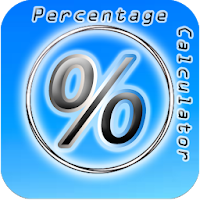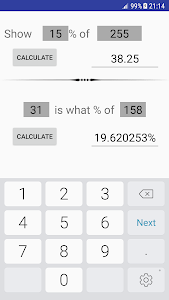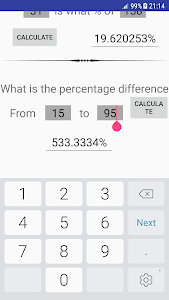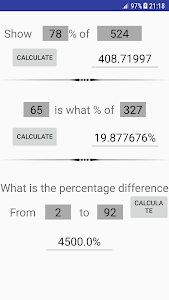[APP] [FREE] My Percentage Calculator

Developers, post your apps here
Forum rules
Read before posting! One topic per app/game. The title should start with, for example, [APP] [FREE] if the app is free.
BeshevGames
Newbie
Posts: 44
Joined: Fri Jul 17, 2015 7:18 pm

[APP] [FREE] My Percentage CalculatorDownload: Google PlayA simple percentage calculator that calculates percents and percentages with 3 main functions:

1. Show result from a percentage from a given number.
2. See a given number is what percentage from another number.
3. See the percentage difference from two given numbers.

This is a simple app to help you with your daily math problems.

It can also be used to calculate a tip in a restaurant.

"In mathematics, a percentage is a number or ratio expressed as a fraction of 100. It is often denoted using the percent sign, "%", or the abbreviations "pct.", "pct"; sometimes the abbreviation "pc" is also used. A percentage is a dimensionless number (pure number)."
-Wikipedia